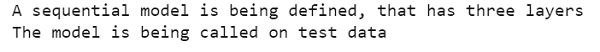# When should a sequential model be used with Tensorflow in Python? Give an example

PythonServer Side ProgrammingProgramming

#### Beyond Basic Programming - Intermediate Python

Most Popular

36 Lectures 3 hours

#### Practical Machine Learning using Python

Best Seller

91 Lectures 23.5 hours

#### Practical Data Science using Python

22 Lectures 6 hours

A sequential model is relevant when there is a plain stack of layers. In this stack, every layer has exactly one input tensor and one output tensor. It is not appropriate when the model has multiple inputs or multiple outputs. It is not appropriate when the layers need to be shared. It is not appropriate when the layer has multiple inputs or multiple outputs. It is not appropriate when a non-linear architecture is required.

Tensorflow is a machine learning framework that is provided by Google. It is an open−source framework used in conjunction with Python to implement algorithms, deep learning applications and much more. It is used in research and for production purposes. It has optimization techniques that help in performing complicated mathematical operations quickly. This is because it uses NumPy and multi−dimensional arrays. These multi-dimensional arrays are also known as ‘tensors’.

Keras was developed as a part of research for the project ONEIROS (Open ended Neuro−Electronic Intelligent Robot Operating System). Keras is a deep learning API, which is written in Python. It is a high−level API that has a productive interface that helps solve machine learning problems. It runs on top of Tensorflow framework. It was built to help experiment in a quick manner. It is highly scalable, and comes with cross platform abilities. This means Keras can be run on TPU or clusters of GPUs. Keras models can also be exported to run in a web browser or a mobile phone as well.

Keras is already present within the Tensorflow package. It can be accessed using the below line of code.

import tensorflow
from tensorflow import keras

We are using the Google Colaboratory to run the below code. Google Colab or Colaboratory helps run Python code over the browser and requires zero configuration and free access to GPUs (Graphical Processing Units). Colaboratory has been built on top of Jupyter Notebook.

Let us see an example to define a sequential model with Tensorflow, including Keras −

## Example

import tensorflow as tf
from tensorflow import keras
from tensorflow.keras import layers
print("A sequential model is being defined, that has three layers")
model = keras.Sequential(
[
layers.Dense(2, activation="relu", name="layer_1"),
layers.Dense(3, activation="relu", name="layer_2"),
layers.Dense(4, name="layer_3"),
]
)
print("The model is being called on test data")
x = tf.ones((2, 2))
y = model(x)

## OutputA sequenital model is being defined, that has three layers
The model is being called on test data
The layers are
[<tensorflow.python.keras.layers.core.Dense object at 0x7fe921aaf7b8>, <tensorflow.python.keras.layers.core.Dense object at 0x7fe921a6d898>, <tensorflow.python.keras.layers.core.Dense object at 0x7fe921a6dc18>]

## Explanation

• The required packages are imported and aliased.

• A sequential model is created using the ‘sequential’ method present in Keras.

• This model is called on test data.

• The details about the layers of the model are displayed on the console.